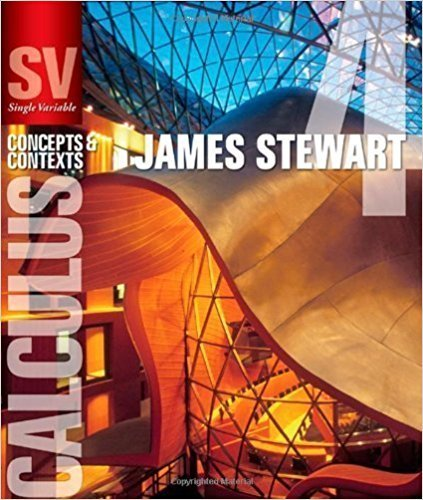×
×

# Solutions for Chapter 7.6: PREDATOR-PREY SYSTEMS## Full solutions for Single Variable Calculus: Concepts and Contexts (Stewart's Calculus Series) | 4th Edition

ISBN: 9780495559726Solutions for Chapter 7.6: PREDATOR-PREY SYSTEMS

Solutions for Chapter 7.6
4 5 0 428 Reviews
14
2
##### ISBN: 9780495559726

Chapter 7.6: PREDATOR-PREY SYSTEMS includes 12 full step-by-step solutions. Single Variable Calculus: Concepts and Contexts (Stewart's Calculus Series) was written by and is associated to the ISBN: 9780495559726. Since 12 problems in chapter 7.6: PREDATOR-PREY SYSTEMS have been answered, more than 20138 students have viewed full step-by-step solutions from this chapter. This expansive textbook survival guide covers the following chapters and their solutions. This textbook survival guide was created for the textbook: Single Variable Calculus: Concepts and Contexts (Stewart's Calculus Series), edition: 4.

Key Calculus Terms and definitions covered in this textbook
• Angle between vectors

The angle formed by two nonzero vectors sharing a common initial point

• Back-to-back stemplot

A stemplot with leaves on either side used to compare two distributions.

• Circle

A set of points in a plane equally distant from a fixed point called the center

• Coefficient matrix

A matrix whose elements are the coefficients in a system of linear equations

• Common difference

See Arithmetic sequence.

• Completing the square

A method of adding a constant to an expression in order to form a perfect square

• Control

The principle of experimental design that makes it possible to rule out other factors when making inferences about a particular explanatory variable

• Explanatory variable

A variable that affects a response variable.

• Graph of an equation in x and y

The set of all points in the coordinate plane corresponding to the pairs x, y that are solutions of the equation.

• Infinite sequence

A function whose domain is the set of all natural numbers.

• Leaf

The final digit of a number in a stemplot.

• Least-squares line

See Linear regression line.

• Newton’s law of cooling

T1t2 = Tm + 1T0 - Tm2e-kt

• Open interval

An interval that does not include its endpoints.

• Paraboloid of revolution

A surface generated by rotating a parabola about its line of symmetry.

• Phase shift

See Sinusoid.

• Reciprocal identity

An identity that equates a trigonometric function with the reciprocal of another trigonometricfunction.

• Symmetric difference quotient of ƒ at a

ƒ(x + h) - ƒ(x - h) 2h

• Term of a polynomial (function)

An expression of the form anxn in a polynomial (function).

• Variation

See Power function.

×